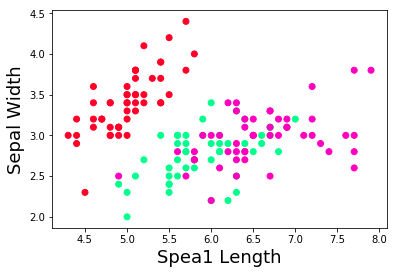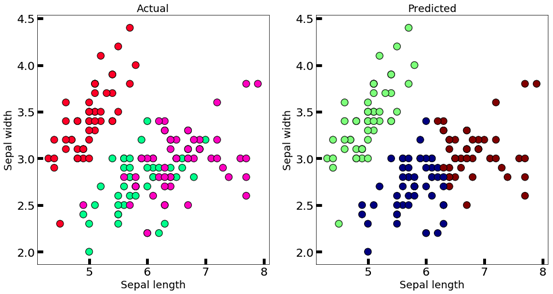### Python K-means Clustering PREDICTING IRIS FLOWER SPECIES

PREDICTING IRIS FLOWER SPECIES WITH K-MEANS CLUSTERING

Clustering is an unsupervisedlearning method that allows us to group set of objects based on similar characteristics. In general, it can help you find meaningful structure among your data, group similar data together and discover underlying patterns.

One of the most common clustering methods is K-means algorithm. The goal of this algorithm isto partition the data into set such that the total sum of squared distances from each point to the mean point of the cluster is minimized.

K means works through the following iterative process:

1.    Pick a value for k (the number of clusters to create)

2.   Initialize k %u2018centroids%u2019 (starting points) in your data

3.   Create your clusters. Assign each point to the nearest centroid.

4.   Make your clusters better. Move each centroid to the center of its cluster.

5.   Repeat steps 3%u20134 until your centroids converge.

How to apply it?

For the following example, I am going to use the Iris data set of scikit learn. This data consists of 50 samples from each of three species of Iris (Iris setosa, Iris virginica and Iris versicolor). It has four features from each sample: length and width of sepals and petals.

1.    To start let%u2019s import the following libraries.

from sklearn import datasetsimport matplotlib.pyplot as pltimport pandas as pdfrom sklearn.cluster import KMeans

3. Define your target and predictors.

X = iris.data[:, :2]y = iris.target

4. Let%u2019s have a look at our data through a scatter plot.

plt.scatter(X[:,0], X[:,1], c=y, cmap='gist_rainbow')
plt.xlabel('Spea1 Length', fontsize=18)
plt.ylabel('Sepal Width', fontsize=18)5. Now, let%u2019s instantiate and fit our K means cluster model. We are going to use three clusters and a random state of 21.

km = KMeans(n_clusters = 3, n_jobs = 4, random_state=21)km.fit(X)

6. With the following code you can identify the center points of the data.

centers = km.cluster_centers_print(centers)Output
[[5.77358491 2.69245283][5.006      3.418    ][6.81276596 3.07446809]]

7. Now, let%u2019s compare our original data versus our clustered results using the following code.

#this will tell us to which cluster does the data observations

belong.new_labels = km.labels_# Plot the identified clusters

and compare with the answersfig, axes = plt.subplots(1, 2,

figsize=(16,8))axes.scatter(X[:, 0], X[:, 1], c=y,

cmap='gist_rainbow',edgecolor='k', s=150)axes.scatter(X[:, 0], X[:, 1], c=new_labels, cmap='jet',edgecolor='k', s=150)axes.set_xlabel('Sepal length', fontsize=18)axes.set_ylabel('Sepal width', fontsize=18)axes.set_xlabel('Sepal length', fontsize=18)axes.set_ylabel('Sepal width', fontsize=18)axes.tick_params(direction='in', length=10, width=5, colors='k', labelsize=20)axes.tick_params(direction='in', length=10, width=5, colors='k', labelsize=20)axes.set_title('Actual', fontsize=18)axes.set_title('Predicted', fontsize=18)Here is a list of the main advantages and disadvantages of this algorithm.

K-Means is simple and computationally efficient.

It is very intuitive and their results are easy to visualize.

K-Means is highly scale dependent and is not suitable for data of varying shapes and densities.

Evaluating results is more subjective. It requires much more human evaluation than trusted metrics.

...

Happy Pythoning......!!#### More Articles of Aditi Kothiyal:

Name Views Likes
Python PyCaret How to optimize the probability threshold % in binary classification 2069 0
Python K-means Predicting Iris Flower Species 1322 2
Python PyCaret How to ignore certain columns for model building 2624 0
Python PyCaret Experiment Logging 680 0
Python PyWin32 Open a File in Excel 941 0
Python Guppy GSL Introduction 219 2
Python Usage of Guppy With Example 1100 2
Python Naive Bayes Tutorial 552 2
Python Guppy Recent Memory Usage of a Program 892 2
Python K-Means Clustering Applications 332 2
Python Random Forest Algorithm Decision Trees 439 0
Python K-means Clustering PREDICTING IRIS FLOWER SPECIES 457 1
Python Random Forest Algorithm Bootstrap 476 0
Python PyCaret Util Functions 441 0
Python K-means Music Genre Classification 1763 1
Python PyWin Attach an Excel file to Outlook 1541 0
Python Guppy GSL Document and Test Example 248 2
Python Random Forest Algorithm Bagging 386 0
Python PyWin32 Getting Started PyWin32 602 0
Python Naive Bayes in Machine Learning 374 2
Python PyCaret How to improve results from hyperparameter tuning by increasing "n_iter" 1723 0
Python PyCaret Getting Started with PyCaret 2.0 356 1
Python PyCaret Tune Model 1325 1
Python PyCaret Create your own AutoML software 320 0
Python PyCaret Intoduction to PyCaret 296 1
Python PyCaret Compare Models 2696 1
Python PyWin Copying Data into Excel 1153 0
Python Guppy Error: expected function body after function declarator 413 2
Python Coding Random forest classifier using xgBoost 247 0
Python PyCaret How to tune "n parameter" in unsupervised experiments 658 0
Python PyCaret How to programmatically define data types in the setup function 1403 0
Python PyCaret Ensemble Model 805 1
Python Random forest algorithm Introduction 227 0
Python k-means Clustering Example 337 1
Python PyCaret Plot Model 1243 1
Python Hamming Distance 715 0
Python Understanding Random forest algorithm 311 0
Python PyCaret Sort a Dictionary by Keys 244 0
Python Coding Random forest classifier using sklearn 340 0
Python Guppy Introduction 368 2
Python How to use Guppy/Heapy for tracking down Memory Usage 1069 2
Python AdaBoost Summary and Conclusion 232 1
Python PyCaret Create Model 365 1
Python k -means Clusturing Introduction 325 2
Python k-means Clustering With Example 348 2